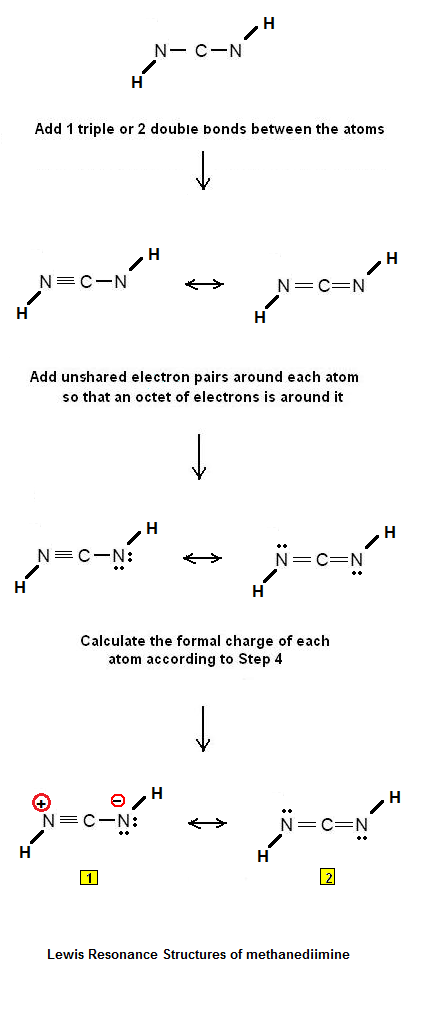Construction of the Lewis electron dot structure of CN2H2 | Chemistry Net

# Construction of the Lewis electron dot structure of CN2H2

A simple and automatic procedure for writing Lewis structures is given in a previous article entitled “Lewis Structures and the Octet Rule”. Relevant worked examples were given in the following articles: Examples #1, #2, #3 , #4, #5, #6,  #7#8, #9, #10, #11, #12, #13, #14 #15, #16, #17, #18, #19, #20, #21, #22,  #23,  #24, #25, #26, #27, #28, #29, #30, #31, #32, #33, #34 and #35.

Let us consider the case of  methanediimine  CN2H2  (HNCNH)

Step 1: Connect the atoms with single bonds.Fig.1: Connect the methanediimine atoms with single bonds

Step 2: Calculate the # of electrons in π bonds (multiple bonds) using  formula (1)

Where n in this case is 3 since CN2H2 has 3 non-hydrogen atoms.
Where V = 5*2 + 4 + 1*2 = 16
Therefore, P = 6n + 2 – V = 6 *3 + 2 – 16 = 4     Therefore, there are either 2 double bonds or 1 triple  bond.

Step 3 & 4: The Lewis electron dot structures of  CN2H2  are as follows:Fig. 2: Resonance Structures of methanediimine

Structure #2 is the most stable Lewis structure because there is an octet of electrons around each atom and there is no charge separation.